#### You may also like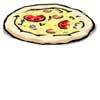### Pizza Portions

My friends and I love pizza. Can you help us share these pizzas equally?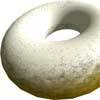### Doughnut

How can you cut a doughnut into 8 equal pieces with only three cuts of a knife?### Pies

Grandma found her pie balanced on the scale with two weights and a quarter of a pie. So how heavy was each pie?

# Fraction Lengths

##### Age 7 to 11Challenge Level

Here are some lengths, which could be made out of connecting cubes or strips of coloured paper/card: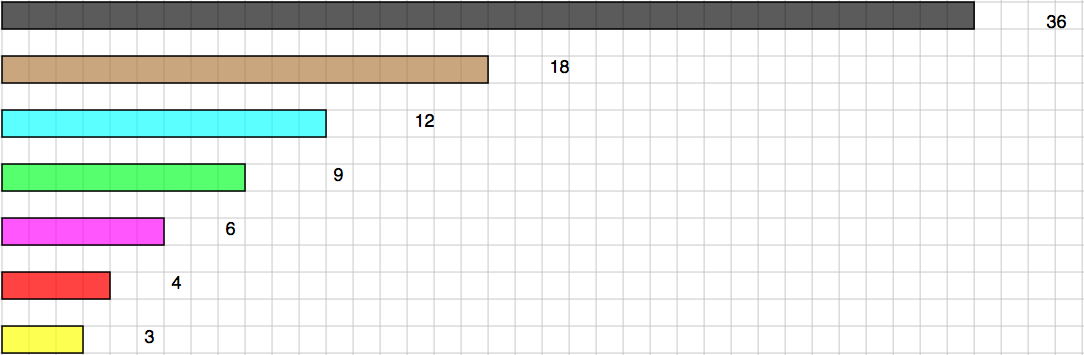To start with, the black will be counted as ONE so that the brown one is $\frac{1}{2}$, the blue one is $\frac{1}{3}$, etc.

Using different combinations, put them together to equal the length of the black, which is 36 long.
For example, if you were to choose the brown, blue and magenta (pink) you could write them down as the $\frac{1}{2}$, $\frac{1}{3}$, $\frac{1}{6}$

So we would have:   $\frac{1}{2} + \frac{1}{3} + \frac{1}{6} = 1$

- - - - - - - - - - - - - - - - - -

#### MOVING ON

Choose any four of the strips, except the black one, and put them together.
Now, compare them with the black.

Here are two examples to start you off. Have a go and find as many different fours as you can.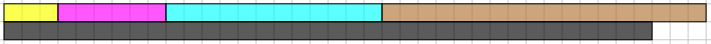Using a 3, 6, 12 and an 18 makes 1$\frac{1}{12}$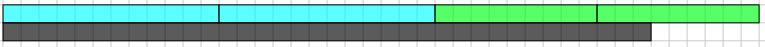Using two 12s and two 9s makes 1$\frac{1}{6}$

- - - - - - - - - - - - - - - - - -

#### GOING EVEN FURTHER

Now the light blue strip is the ONE (1).
Use the same fours that you chose before but this time, compare them with the light blue strip instead of the black

Here are the examples used above, but this time compared with a light blue: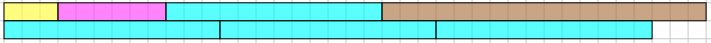Comparing these four to the light blue it makes 3$\frac{1}{4}$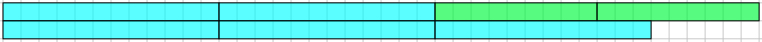Comparing these four to the light blue it makes 3$\frac{1}{2}$

Now you go ahead with the fours that you have chosen.

- - - - - - - - - - - - - - - - - -

What can you say about the results you got when comparing your fours with black and comparing them with the light blue?